# M5-S3: Full-flight Projectile Motion - Maximum Height, Time of Flight and Range

• ### Apply the modelling of projectile motion to quantitatively derive the relationships between the following variables:

• maximum height
• time of flight
• final velocity
• horizontal range of the projectile

### Maximum Height

• The maximum height of an object’s projectile motion is the point at which it reaches the highest vertical displacement during the projectile motion. In other words, it is the maximum point of the parabolic path.
• Maximum height is dictated by initial launch height and initial vertical velocity. It is not affected by initial horizontal velocity.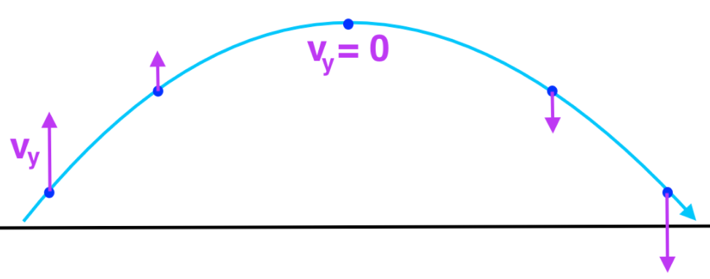• At maximum height, vertical velocity equals zero. Thus, the instantaneous velocity at this point equals to the horizontal velocity.
• Calculating maximum height is usually associated with the following rectilinear equation:

v_y^2=u_y^2+2a_ys

### Time of Flight

• Time of flight refers to the duration of the entire projectile motion: from the time of launch to the time when it reaches its destination.
• Time of flight is dictated by initial vertical velocity. It is not affected by initial horizontal velocity.
• Calculating time of flight is usually associated with the following equation:

s=u_yt+1/2a_yt^2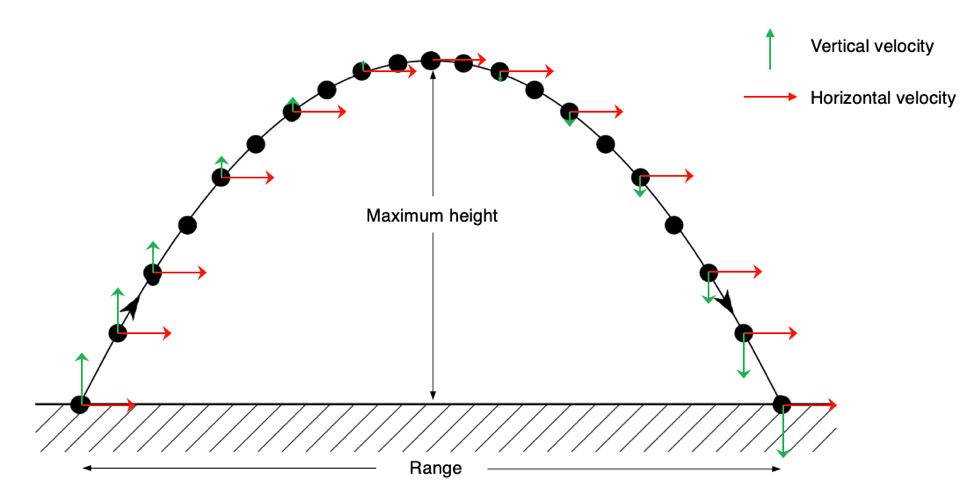### Range

• At completion of motion, the total horizontal displacement of the motion is referred to as the range.
• The range or horizontal displacement is dictated by the object’s initial horizontal velocity and time of flight. As a result, it is indirectly affected by initial vertical velocity.
• Since horizontal velocity is never concerned with acceleration, range or horizontal displacement is calculated using the simple relationship between distance, speed or time:

Deltax=u_x*t_(flight)

## Practice Question 1

A projectile is launched at 100 ms-1 at an elevation of 45º relative to the ground.

(a) Calculate the vertical component of its velocity.

u_y=usintheta

u_y=100sin45^o

u_y=70.7  ms^-1

(b) Calculate the time of flight of the projectile.

s=u_yt+1/2a_yt^2

At the completion of projectile motion, the vertical displacement s is 0:

0=(70.7)t+1/2(-9.8)t^2

0=70.7t-4.9t^2

0=t(70.7-4.9t)

t=0 or t=14.4 seconds

Time of flight = 14.4 seconds. t = 0 corresponds to when the object is about to be launched.

(c) Calculate the maximum height of the projectile above its launch position.

v_y^2=u_y^2+2a_ys

At maximum height, vertical velocity = 0:

(0)^2=(70.7)^2+2(-9.8)s

s=(70.7)^2/(2xx9.8)

s=255

Maximum height = 255 m above ground

(d) Calculate the range of the projectile.

Deltax=u_x*t_(flight)

In order to calculate the range, initial horizontal velocity is required.

u_x=ucostheta

u_x=100cos45^o

u_x=70.7  ms^-1
Calculate range:

Deltax=(70.7)*(14.4)

Deltax=1020 m

This is what the projectile motion looks like: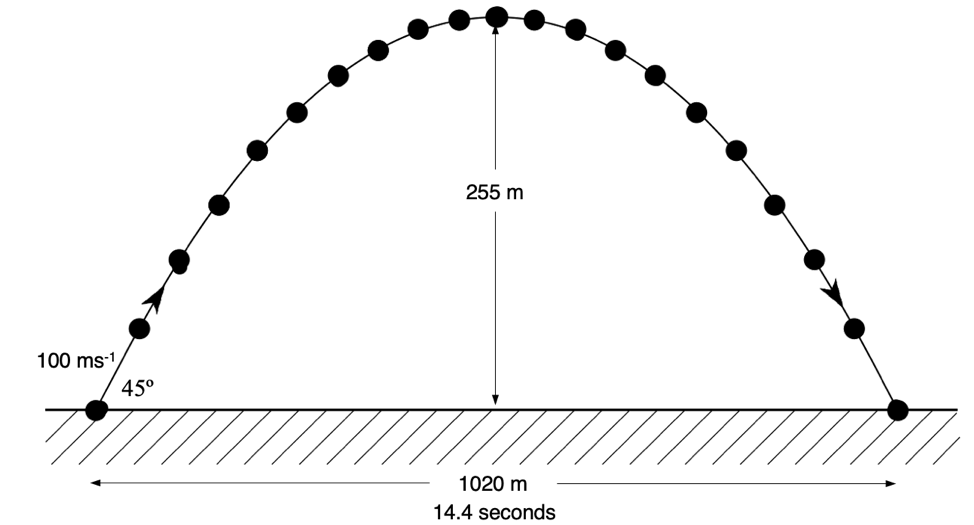## Practice Question 2

A cricket ball was thrown with an initial velocity, U = 15.0 ms-1 at an angle of elevation of 35º, as shown in the diagram below.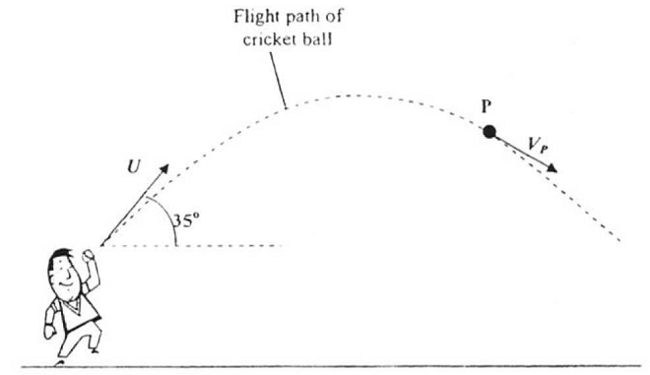At point P, in the flight, a remote sensor measured the vertical component of the velocity of the ball to be 6.7 ms-1 downwards.

Calculate the magnitude and direction of the instantaneous velocity. Vp, of the cricket ball when it is at point, P, in the flight.

Since we are given the vertical velocity at point P, we will need to find the horizontal component to find Vp﻿.

The horizontal component remains constant throughout projectile motion. The initial horizontal velocity is:

u_x=15cos35º

u_x=12.3 m/s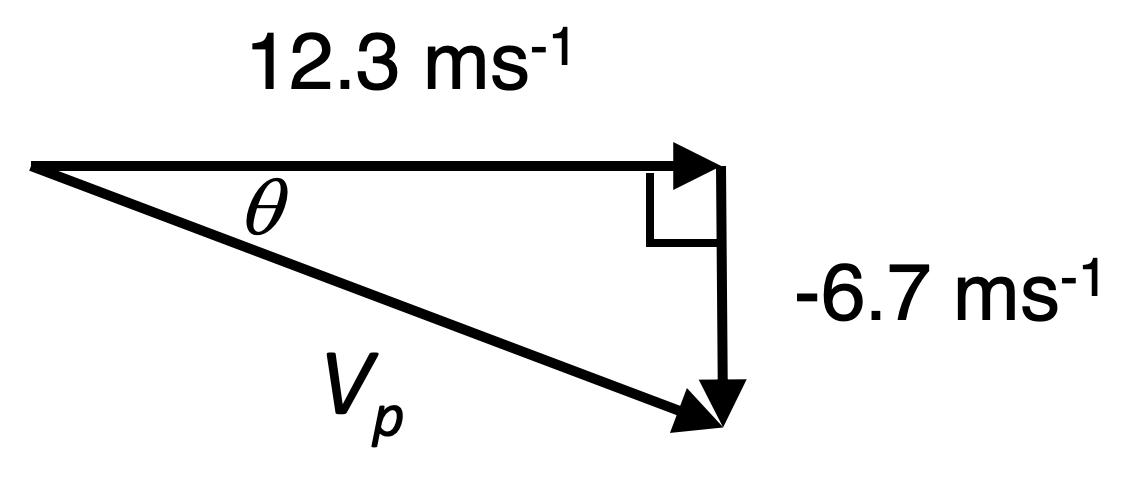Using Pythagoras's theorem to calculate velocity at point P:

V=sqrt(12.3^2+(-6.7)^2)

V=14.0 m/s

To find the direction, we will need to use trigonometry:

tantheta=6.7/12.3

theta=tan^-1(6.7/12.3)

theta=28.6º

Therefore, the the cricket ball is travelling at 14.0 m/s at an angle of 28.6º below the horizontal.